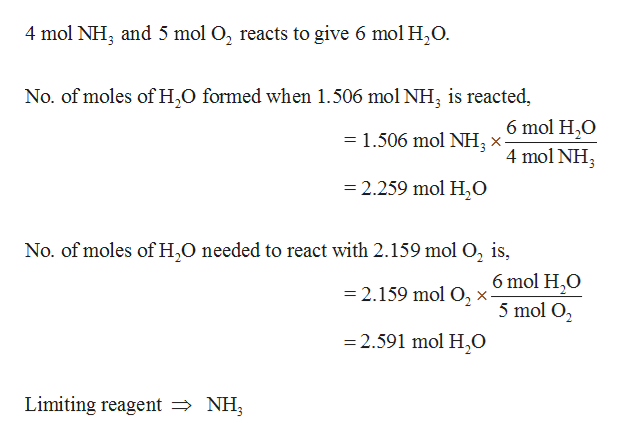# 4 NH3 (g) + 5 O2 (g) → 4 NO (g) + 6 H2O (g)What is the maximum mass of water that can be produced by the reaction of 25.64 g of ammonia and 69.0998 g of oxygen?

Question
21 views

4 NH3 (g) + 5 O2 (g) → 4 NO (g) + 6 H2O (g)

What is the maximum mass of water that can be produced by the reaction of 25.64 g of ammonia and 69.0998 g of oxygen?

check_circle

Step 1

Number of moles of each reactant is calculated.

Step 2

Determine limitin...help_outlineImage Transcriptionclose4 mol NH3 and 5 mol O2 reacts to give 6 mol H,20 No. of moles of H2O formed when 1.506 mol NH, is reacted, 6 mol H,O =1.506 mol NH3 x- 4 mol NH3 2.259 mol H20 No. of moles of H,O needed to react with 2.159 mol O2 is, 6 mol H,O 5 mol O2 =2.159 mol O2 x- 2.591 mol H0 Limiting reagent NH fullscreen

### Want to see the full answer?

See Solution

#### Want to see this answer and more?

Solutions are written by subject experts who are available 24/7. Questions are typically answered within 1 hour.*

See Solution
*Response times may vary by subject and question.
Tagged in

### General Chemistry